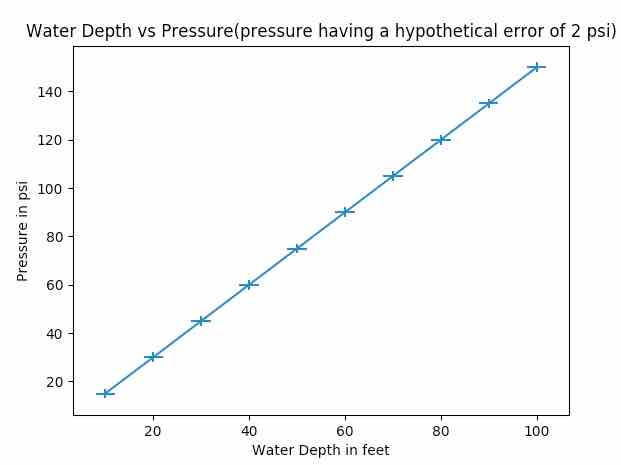# Drawing A Plot With Error Bars Using Python Matplotlib

## Overview:

• Error bars indicate how much each data point in a plot deviates from the actual value.

• Error bars display the standard deviation of the distribution while the actual plot depicts the shape of the distribution.

• For graphs present in the research papers it is a necessary to display the error value along with the data points which depicts the amount of uncertainty present in the data.

• The function matplotlib.pyplot.errorbar() plots given x values and y values in a graph and marks the error or standard deviation of the distribution on each point.

## Example:

 import numpy as np import matplotlib.pyplot as plot   # Depth data xData        = np.arange(10, 110, 10)   multiplesOf  = 15 dataCount    = 10   # Water pressure data yData        = list(range(multiplesOf, (dataCount+1)*multiplesOf, multiplesOf))   # Set error values for X axis and Y axis data xerror         = 2 yerror         = 2   # Draw the plot with error bars plot.errorbar(xData, yData, xerr=xerror, yerr=yerror, errorevery=1, markeredgewidth=10)   # Set X axis label for the errorbar graph plot.xlabel('Water Depth in feet')   # Set Y axis label for the errorbar graph plot.ylabel('Pressure in psi')   # Set Title for the errorbar graph plot.title('Water Depth vs Pressure(pressure having a hypothetical error of 2 psi)')   # Display the errorbar graph plot.show()

## Output: